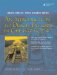# C++ Standard Library Strings

Our early (pre-Qt) examples will make use of C++ Standard Library strings (see Appendix B). Standard Library strings have many disadvantages when compared to QStrings, but they are easy to use and have a similar public interface that includes many functions to construct and modify strings.

Example 1.14 demonstrates its basic usage.

Example 1.14. src/generic/stlstringdemo.cpp

 ```#include #include int main() { using namespace std; string s1("This "), s2("is a "), s3("string."); s1 += s2; <-- 1 string s4 = s1 + s3; cout << s4 << endl; string s5("The length of that string is: "); cout << s5 << s4.length << " characters." << endl; cout << "Enter a sentence: " << endl; getline(cin, s2); cout << "Here is your sentence: " << s2 << endl; cout << "The length of it was: " << s2.length() << endl; return 0; }```   (1)concatenation

Here is the compile and run:

```src/generic> g++ -ansi -pedantic -Wall stlstringdemo.cpp
src/generic> ./a.out
This is a string.
The length of that string is 17
Enter a sentence:
20 years hard labour
20 years hard labour
The length of it was: 20
src/generic>
```

Observe that we used the getline(istream, string) function to take a string from standard input stream. We will learn more about input from streams in the following section.

### StreamsAn Introduction to Design Patterns in C++ with Qt 4
ISBN: 0131879057
EAN: 2147483647
Year: 2004
Pages: 268